Chi Distribution

#Chi Distribution

Chi is the distribution of the square root of a random variable distributed as Chi Square. The Chi Distribution does not commonly apply to a wide range of cases, and care must be taken to ensure that the circumstances are appropriate when choosing the Chi Distribution.

The Chi Distribution is defined for all positive values of x. It has two parameters, n and sigma, both of which must be positive. In addition, n must be an integer. The distribution is: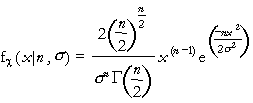where x>0, n=1,2,..., and sigma>0. The gamma function,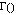, is a generalization of the factorial function. Factorials are only defined for integers, but the gamma function can have any number for an argument. If n is an integer,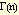= (n-1)!.

The equations for the mean and variance are: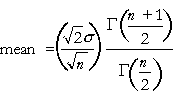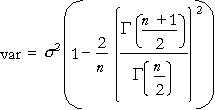Versions: DPL Professional, DPL Enterprise, DPL Portfolio